Open in App
Not now

# Python | Pandas DataFrame.astype()

• Last Updated : 25 Jul, 2019

Python is a great language for doing data analysis, primarily because of the fantastic ecosystem of data-centric python packages. Pandas is one of those packages and makes importing and analyzing data much easier.

`DataFrame.astype()` method is used to cast a pandas object to a specified dtype. `astype()` function also provides the capability to convert any suitable existing column to categorical type.

`DataFrame.astype()` function comes very handy when we want to case a particular column data type to another data type. Not only that but we can also use a Python dictionary input to change more than one column type at once. The key label in dictionary is corresponding to the column name and the values label in the dictionary is corresponding to the new data types we want the columns to be of.

Syntax: DataFrame.astype(dtype, copy=True, errors=’raise’, **kwargs)

Parameters:
dtype : Use a `numpy.dtype `or Python type to cast entire pandas object to the same type. Alternatively, use {col: dtype, …}, where col is a column label and dtype is a `numpy.dtype` or Python type to cast one or more of the DataFrame’s columns to column-specific types.
copy : Return a copy when copy=True (be very careful setting copy=False as changes to values then may propagate to other pandas objects).

errors : Control raising of exceptions on invalid data for provided dtype.
raise : allow exceptions to be raised
ignore : suppress exceptions. On error return original object

kwargs :keyword arguments to pass on to the constructor

Returns: casted : type of caller

Example #1: Convert the Weight column data type.

 `# importing pandas as pd``import` `pandas as pd`` ` `# Making data frame from the csv file``df ``=` `pd.read_csv(``"nba.csv"``)`` ` `# Printing the first 10 rows of ``# the data frame for visualization`` ` `df[:``10``]`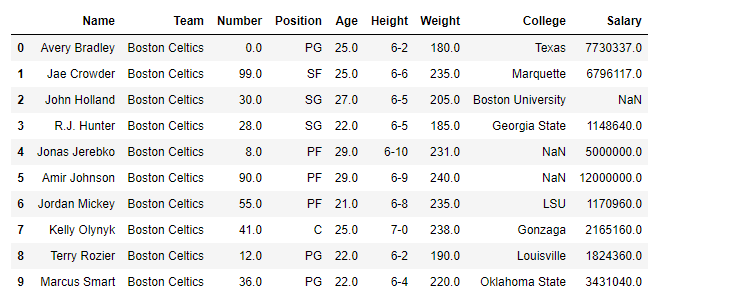As the data have some “nan” values so, to avoid any error we will drop all the rows containing any `nan `values.

 `# drop all those rows which ``# have any 'nan' value in it.``df.dropna(inplace ``=` `True``)`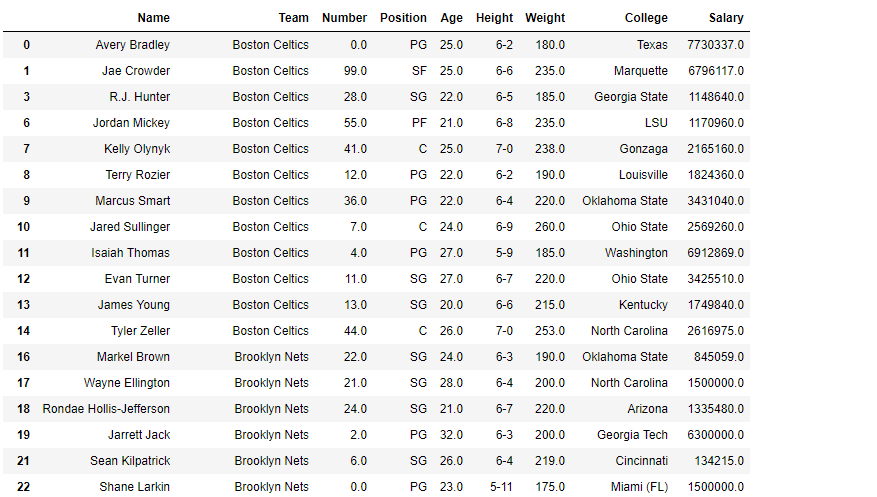`# let's find out the data type of Weight column``before ``=` `type``(df.Weight[``0``])`` ` `# Now we will convert it into 'int64' type.``df.Weight ``=` `df.Weight.astype(``'int64'``)`` ` `# let's find out the data type after casting``after ``=` `type``(df.Weight[``0``])`` ` `# print the value of before``before`` ` `# print the value of after``after`

Output: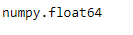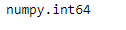`# print the data frame and see``# what it looks like after the change``df`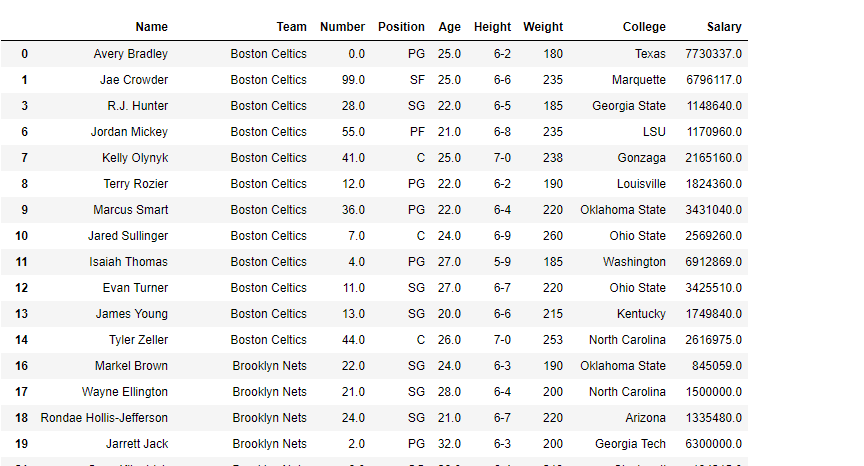Example #2: Change the data type of more than one column at once

Change the `Name `column to categorical type and `Age `column to int64 type.

 `# importing pandas as pd``import` `pandas as pd`` ` `# Making data frame from the csv file``df ``=` `pd.read_csv(``"nba.csv"``)`` ` `# Drop the rows with 'nan' values``df ``=` `df.dropna()`` ` `# print the existing data type of each column``df.info()`

Output: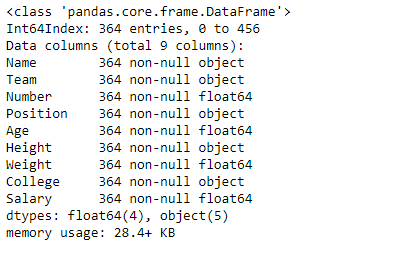Now let’s change both the columns data type at once.

 `# Passed a dictionary to astype() function ``df ``=` `df.astype({``"Name"``:``'category'``, ``"Age"``:``'int64'``})`` ` `# Now print the data type ``# of all columns after change``df.info()`

Output: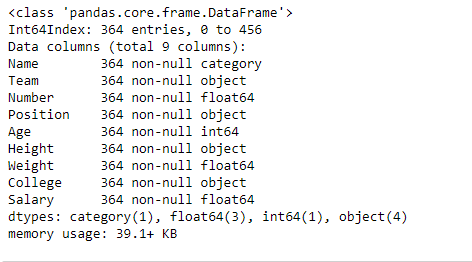`# print the data frame``# too after the change``df`

Output: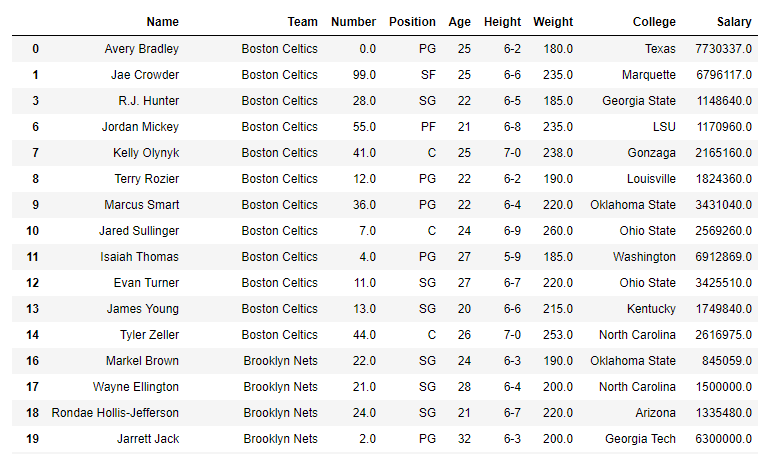My Personal Notes arrow_drop_up